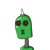# 2. The following numbers are obviously not perfect squares. Give reason.6) 1057(ii) 23453(ii) 7928(v) 2222

2. The following numbers are obviously not perfect squares. Give reason.
6) 1057
(ii) 23453
(ii) 7928
(v) 222222
(v) 64000
(vi) 89722
(vii) 222000 (vi) 505050​

### 1 thought on “2. The following numbers are obviously not perfect squares. Give reason.<br />6) 1057<br />(ii) 23453<br />(ii) 7928<br />(v) 2222”

1.Step-by-step explanation:

Ncert solutions

Mathematics

Science

Chapters in NCERT Solutions – Mathematics , Class 8

Exercises in Squares and Square Roots

Question 2

Q2) The following numbers are obviously not perfect squares. Give reasons.

(i) 1057

(ii) 23453

(iii) 7928

(iv) 222222

(v) 64000

(vi) 89722

(vii) 222000

(viii) 505050

Solution

Transcript

Solution:

(i) Since, perfect square numbers contain their unit’s place digit 1, 4, 5, 6, 9 and even numbers of 0. Therefore 1057 is not a perfect square because its unit’s place digit is 7.

(ii) Since, perfect square numbers contain their unit’s place digit 0, 1, 4, 5, 6, 9 and even number of 0. Therefore 23453 is not a perfect square because its unit’s place digit is 3.

(iii) Since, perfect square numbers contain their unit’s place digit 0, 1, 4, 5, 6, 9 and even number of 0. Therefore 7928 is not a perfect square because its unit’s place digit is 8.

(iv) Since, perfect square numbers contain their unit’s place digit 0, 1, 4, 5, 6, 9 and even number of 0. Therefore 222222 is not a perfect square because its unit’s place digit is 2.

(v) Since, perfect square numbers contain their unit’s place digit 0, 1, 4, 5, 6, 9 and even number of 0. Therefore 64000 is not a perfect square because its unit’s place digit is single 0.

(vi) Since, perfect square numbers contain their unit’s place digit 0, 1, 4, 5, 6, 9 and even number of 0. Therefore 89722 is not a perfect square because its unit’s place digit is 2.

(vii) Since, perfect square numbers contain their unit’s place digit 0, 1, 4, 5, 6, 9 and even number of 0. Therefore 222000 is not a perfect square because its unit’s place digit is triple 0.

(viii) Since, perfect square numbers contain their unit’s place digit 0, 1, 4, 5, 6, 9 and even number of 0. Therefore 505050 is not a perfect square because its unit’s place digit is 0.